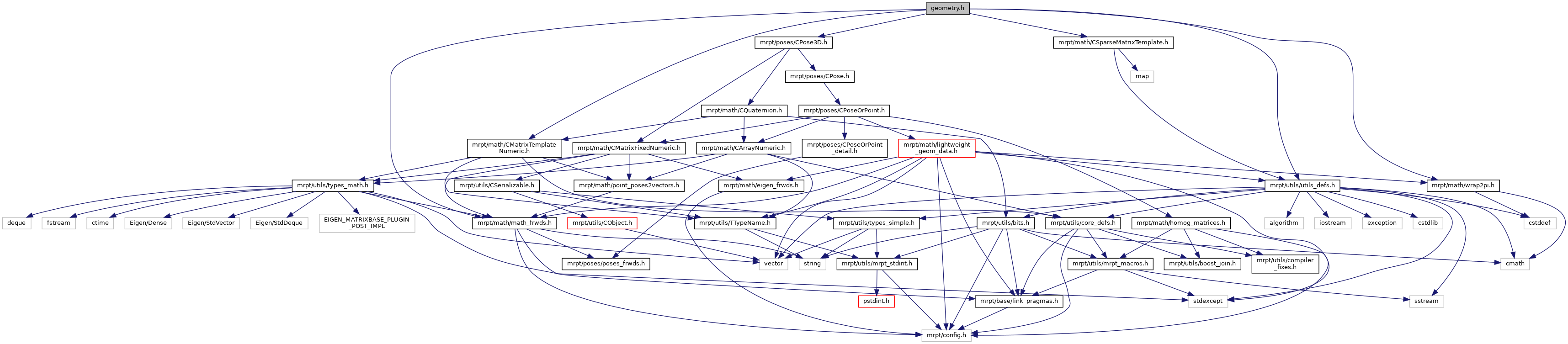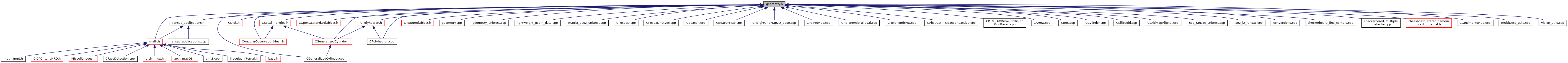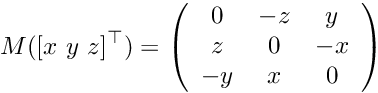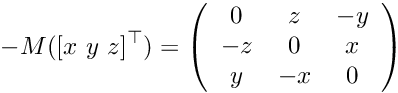Main MRPT website > C++ reference for MRPT 1.5.7
geometry.h File Reference
#include <mrpt/utils/utils_defs.h>
#include <mrpt/math/CMatrixTemplateNumeric.h>
#include <mrpt/poses/CPose3D.h>
#include <mrpt/math/CSparseMatrixTemplate.h>
#include <mrpt/math/math_frwds.h>
#include <mrpt/math/wrap2pi.h>
Include dependency graph for geometry.h:This graph shows which files directly or indirectly include this file:Go to the source code of this file.

## Classes

class  mrpt::math::TPolygonWithPlane
Slightly heavyweight type to speed-up calculations with polygons in 3D. More...

## Namespaces

mrpt
This is the global namespace for all Mobile Robot Programming Toolkit (MRPT) libraries.

mrpt::math
This base provides a set of functions for maths stuff.

## Functions

Simple intersection operations, relying basically on geometrical operations.
bool BASE_IMPEXP mrpt::math::intersect (const TSegment3D &s1, const TSegment3D &s2, TObject3D &obj)
Gets the intersection between two 3D segments. More...

bool BASE_IMPEXP mrpt::math::intersect (const TSegment3D &s1, const TPlane &p2, TObject3D &obj)
Gets the intersection between a 3D segment and a plane. More...

bool BASE_IMPEXP mrpt::math::intersect (const TSegment3D &s1, const TLine3D &r2, TObject3D &obj)
Gets the intersection between a 3D segment and a 3D line. More...

bool mrpt::math::intersect (const TPlane &p1, const TSegment3D &s2, TObject3D &obj)
Gets the intersection between a plane and a 3D segment. More...

bool BASE_IMPEXP mrpt::math::intersect (const TPlane &p1, const TPlane &p2, TObject3D &obj)
Gets the intersection between two planes. More...

bool BASE_IMPEXP mrpt::math::intersect (const TPlane &p1, const TLine3D &p2, TObject3D &obj)
Gets the intersection between a plane and a 3D line. More...

bool mrpt::math::intersect (const TLine3D &r1, const TSegment3D &s2, TObject3D &obj)
Gets the intersection between a 3D line and a 3D segment. More...

bool mrpt::math::intersect (const TLine3D &r1, const TPlane &p2, TObject3D &obj)
Gets the intersection between a 3D line and a plane. More...

bool BASE_IMPEXP mrpt::math::intersect (const TLine3D &r1, const TLine3D &r2, TObject3D &obj)
Gets the intersection between two 3D lines. More...

bool BASE_IMPEXP mrpt::math::intersect (const TLine2D &r1, const TLine2D &r2, TObject2D &obj)
Gets the intersection between two 2D lines. More...

bool BASE_IMPEXP mrpt::math::intersect (const TLine2D &r1, const TSegment2D &s2, TObject2D &obj)
Gets the intersection between a 2D line and a 2D segment. More...

bool mrpt::math::intersect (const TSegment2D &s1, const TLine2D &r2, TObject2D &obj)
Gets the intersection between a 2D line and a 2D segment. More...

bool BASE_IMPEXP mrpt::math::intersect (const TSegment2D &s1, const TSegment2D &s2, TObject2D &obj)
Gets the intersection between two 2D segments. More...

Angle retrieval methods. Methods which use TSegments will automatically use TLines' implicit constructors.
double BASE_IMPEXP mrpt::math::getAngle (const TPlane &p1, const TPlane &p2)
Computes the angle between two planes. More...

double BASE_IMPEXP mrpt::math::getAngle (const TPlane &p1, const TLine3D &r2)
Computes the angle between a plane and a 3D line or segment (implicit constructor will be used if passing a segment instead of a line). More...

double mrpt::math::getAngle (const TLine3D &r1, const TPlane &p2)
Computes the angle between a 3D line or segment and a plane (implicit constructor will be used if passing a segment instead of a line). More...

double BASE_IMPEXP mrpt::math::getAngle (const TLine3D &r1, const TLine3D &r2)
Computes the angle between two 3D lines or segments (implicit constructor will be used if passing a segment instead of a line). More...

double BASE_IMPEXP mrpt::math::getAngle (const TLine2D &r1, const TLine2D &r2)
Computes the angle between two 2D lines or segments (implicit constructor will be used if passing a segment instead of a line). More...

Creation of lines from poses.
void BASE_IMPEXP mrpt::math::createFromPoseX (const mrpt::poses::CPose3D &p, TLine3D &r)
Gets a 3D line corresponding to the X axis in a given pose. More...

void BASE_IMPEXP mrpt::math::createFromPoseY (const mrpt::poses::CPose3D &p, TLine3D &r)
Gets a 3D line corresponding to the Y axis in a given pose. More...

void BASE_IMPEXP mrpt::math::createFromPoseZ (const mrpt::poses::CPose3D &p, TLine3D &r)
Gets a 3D line corresponding to the Z axis in a given pose. More...

void BASE_IMPEXP mrpt::math::createFromPoseAndVector (const mrpt::poses::CPose3D &p, const double(&vector), TLine3D &r)
Gets a 3D line corresponding to any arbitrary vector, in the base given by the pose. More...

void BASE_IMPEXP mrpt::math::createFromPoseX (const TPose2D &p, TLine2D &r)
Gets a 2D line corresponding to the X axis in a given pose. More...

void BASE_IMPEXP mrpt::math::createFromPoseY (const TPose2D &p, TLine2D &r)
Gets a 2D line corresponding to the Y axis in a given pose. More...

void BASE_IMPEXP mrpt::math::createFromPoseAndVector (const TPose2D &p, const double(&vector), TLine2D &r)
Gets a 2D line corresponding to any arbitrary vector, in the base given the given pose. More...

Other line or plane related methods.
bool BASE_IMPEXP mrpt::math::conformAPlane (const std::vector< TPoint3D > &points)
Checks whether this polygon or set of points acceptably fits a plane. More...

bool BASE_IMPEXP mrpt::math::conformAPlane (const std::vector< TPoint3D > &points, TPlane &p)
Checks whether this polygon or set of points acceptably fits a plane, and if it's the case returns it in the second argument. More...

bool BASE_IMPEXP mrpt::math::areAligned (const std::vector< TPoint2D > &points)
Checks whether this set of points acceptably fits a 2D line. More...

bool BASE_IMPEXP mrpt::math::areAligned (const std::vector< TPoint2D > &points, TLine2D &r)
Checks whether this set of points acceptably fits a 2D line, and if it's the case returns it in the second argument. More...

bool BASE_IMPEXP mrpt::math::areAligned (const std::vector< TPoint3D > &points)
Checks whether this set of points acceptably fits a 3D line. More...

bool BASE_IMPEXP mrpt::math::areAligned (const std::vector< TPoint3D > &points, TLine3D &r)
Checks whether this set of points acceptably fits a 3D line, and if it's the case returns it in the second argument. More...

Projections
void mrpt::math::project3D (const TPoint3D &point, const mrpt::poses::CPose3D &newXYpose, TPoint3D &newPoint)
Uses the given pose 3D to project a point into a new base. More...

void mrpt::math::project3D (const TSegment3D &segment, const mrpt::poses::CPose3D &newXYpose, TSegment3D &newSegment)
Uses the given pose 3D to project a segment into a new base. More...

void BASE_IMPEXP mrpt::math::project3D (const TLine3D &line, const mrpt::poses::CPose3D &newXYpose, TLine3D &newLine)
Uses the given pose 3D to project a line into a new base. More...

void BASE_IMPEXP mrpt::math::project3D (const TPlane &plane, const mrpt::poses::CPose3D &newXYpose, TPlane &newPlane)
Uses the given pose 3D to project a plane into a new base. More...

void BASE_IMPEXP mrpt::math::project3D (const TPolygon3D &polygon, const mrpt::poses::CPose3D &newXYpose, TPolygon3D &newPolygon)
Uses the given pose 3D to project a polygon into a new base. More...

void BASE_IMPEXP mrpt::math::project3D (const TObject3D &object, const mrpt::poses::CPose3D &newXYPose, TObject3D &newObject)
Uses the given pose 3D to project any 3D object into a new base. More...

template<class T >
void mrpt::math::project3D (const T &obj, const TPlane &newXYPlane, T &newObj)
Projects any 3D object into the plane's base, using its inverse pose. More...

template<class T >
void mrpt::math::project3D (const T &obj, const TPlane &newXYPlane, const TPoint3D &newOrigin, T &newObj)
Projects any 3D object into the plane's base, using its inverse pose and forcing the position of the new coordinates origin. More...

template<class T >
void mrpt::math::project3D (const std::vector< T > &objs, const mrpt::poses::CPose3D &newXYpose, std::vector< T > &newObjs)
Projects a set of 3D objects into the plane's base. More...

void BASE_IMPEXP mrpt::math::project2D (const TPoint2D &point, const mrpt::poses::CPose2D &newXpose, TPoint2D &newPoint)
Uses the given pose 2D to project a point into a new base. More...

void mrpt::math::project2D (const TSegment2D &segment, const mrpt::poses::CPose2D &newXpose, TSegment2D &newSegment)
Uses the given pose 2D to project a segment into a new base. More...

void BASE_IMPEXP mrpt::math::project2D (const TLine2D &line, const mrpt::poses::CPose2D &newXpose, TLine2D &newLine)
Uses the given pose 2D to project a line into a new base. More...

void BASE_IMPEXP mrpt::math::project2D (const TPolygon2D &polygon, const mrpt::poses::CPose2D &newXpose, TPolygon2D &newPolygon)
Uses the given pose 2D to project a polygon into a new base. More...

void BASE_IMPEXP mrpt::math::project2D (const TObject2D &object, const mrpt::poses::CPose2D &newXpose, TObject2D &newObject)
Uses the given pose 2D to project any 2D object into a new base. More...

template<class T , class CPOSE2D >
void mrpt::math::project2D (const T &obj, const TLine2D &newXLine, T &newObj)
Projects any 2D object into the line's base, using its inverse pose. More...

template<class T , class CPOSE2D >
void mrpt::math::project2D (const T &obj, const TLine2D &newXLine, const TPoint2D &newOrigin, T &newObj)
Projects any 2D object into the line's base, using its inverse pose and forcing the position of the new coordinate origin. More...

template<class T >
void mrpt::math::project2D (const std::vector< T > &objs, const mrpt::poses::CPose2D &newXpose, std::vector< T > &newObjs)
Projects a set of 2D objects into the line's base. More...

Polygon intersections. These operations rely more on spatial reasoning than in raw numerical operations.
bool BASE_IMPEXP mrpt::math::intersect (const TPolygon2D &p1, const TSegment2D &s2, TObject2D &obj)
Gets the intersection between a 2D polygon and a 2D segment. More...

bool BASE_IMPEXP mrpt::math::intersect (const TPolygon2D &p1, const TLine2D &r2, TObject2D &obj)
Gets the intersection between a 2D polygon and a 2D line. More...

bool BASE_IMPEXP mrpt::math::intersect (const TPolygon2D &p1, const TPolygon2D &p2, TObject2D &obj)
Gets the intersection between two 2D polygons. More...

bool mrpt::math::intersect (const TSegment2D &s1, const TPolygon2D &p2, TObject2D &obj)
Gets the intersection between a 2D segment and a 2D polygon. More...

bool mrpt::math::intersect (const TLine2D &r1, const TPolygon2D &p2, TObject2D &obj)
Gets the intersection between a 2D line and a 2D polygon. More...

bool BASE_IMPEXP mrpt::math::intersect (const TPolygon3D &p1, const TSegment3D &s2, TObject3D &obj)
Gets the intersection between a 3D polygon and a 3D segment. More...

bool BASE_IMPEXP mrpt::math::intersect (const TPolygon3D &p1, const TLine3D &r2, TObject3D &obj)
Gets the intersection between a 3D polygon and a 3D line. More...

bool BASE_IMPEXP mrpt::math::intersect (const TPolygon3D &p1, const TPlane &p2, TObject3D &obj)
Gets the intersection between a 3D polygon and a plane. More...

bool BASE_IMPEXP mrpt::math::intersect (const TPolygon3D &p1, const TPolygon3D &p2, TObject3D &obj)
Gets the intersection between two 3D polygons. More...

bool mrpt::math::intersect (const TSegment3D &s1, const TPolygon3D &p2, TObject3D &obj)
Gets the intersection between a 3D segment and a 3D polygon. More...

bool mrpt::math::intersect (const TLine3D &r1, const TPolygon3D &p2, TObject3D &obj)
Gets the intersection between a 3D line and a 3D polygon. More...

bool mrpt::math::intersect (const TPlane &p1, const TPolygon3D &p2, TObject3D &obj)
Gets the intersection between a plane and a 3D polygon. More...

size_t BASE_IMPEXP mrpt::math::intersect (const std::vector< TPolygon3D > &v1, const std::vector< TPolygon3D > &v2, CSparseMatrixTemplate< TObject3D > &objs)
Gets the intersection between two sets of 3D polygons. More...

size_t BASE_IMPEXP mrpt::math::intersect (const std::vector< TPolygon3D > &v1, const std::vector< TPolygon3D > &v2, std::vector< TObject3D > &objs)
Gets the intersection between two sets of 3D polygons. More...

Other intersections
template<class T , class U , class O >
size_t mrpt::math::intersect (const std::vector< T > &v1, const std::vector< U > &v2, CSparseMatrixTemplate< O > &objs)
Gets the intersection between vectors of geometric objects and returns it in a sparse matrix of either TObject2D or TObject3D. More...

template<class T , class U , class O >
size_t mrpt::math::intersect (const std::vector< T > &v1, const std::vector< U > &v2, std::vector< O > objs)
Gets the intersection between vectors of geometric objects and returns it in a vector of either TObject2D or TObject3D. More...

bool BASE_IMPEXP mrpt::math::intersect (const TObject2D &o1, const TObject2D &o2, TObject2D &obj)
Gets the intersection between any pair of 2D objects. More...

bool BASE_IMPEXP mrpt::math::intersect (const TObject3D &o1, const TObject3D &o2, TObject3D &obj)
Gets the intersection between any pair of 3D objects. More...

Distances
double BASE_IMPEXP mrpt::math::distance (const TPoint2D &p1, const TPoint2D &p2)
Gets the distance between two points in a 2D space. More...

double BASE_IMPEXP mrpt::math::distance (const TPoint3D &p1, const TPoint3D &p2)
Gets the distance between two points in a 3D space. More...

double BASE_IMPEXP mrpt::math::distance (const TLine2D &r1, const TLine2D &r2)
Gets the distance between two lines in a 2D space. More...

double BASE_IMPEXP mrpt::math::distance (const TLine3D &r1, const TLine3D &r2)
Gets the distance between two lines in a 3D space. More...

double BASE_IMPEXP mrpt::math::distance (const TPlane &p1, const TPlane &p2)
Gets the distance between two planes. It will be zero if the planes are not parallel. More...

double BASE_IMPEXP mrpt::math::distance (const TPolygon2D &p1, const TPolygon2D &p2)
Gets the distance between two polygons in a 2D space. More...

double BASE_IMPEXP mrpt::math::distance (const TPolygon2D &p1, const TSegment2D &s2)
Gets the distance between a polygon and a segment in a 2D space. More...

double mrpt::math::distance (const TSegment2D &s1, const TPolygon2D &p2)
Gets the distance between a segment and a polygon in a 2D space. More...

double BASE_IMPEXP mrpt::math::distance (const TPolygon2D &p1, const TLine2D &l2)
Gets the distance between a polygon and a line in a 2D space. More...

double mrpt::math::distance (const TLine2D &l1, const TPolygon2D &p2)

double BASE_IMPEXP mrpt::math::distance (const TPolygon3D &p1, const TPolygon3D &p2)
Gets the distance between two polygons in a 3D space. More...

double BASE_IMPEXP mrpt::math::distance (const TPolygon3D &p1, const TSegment3D &s2)
Gets the distance between a polygon and a segment in a 3D space. More...

double mrpt::math::distance (const TSegment3D &s1, const TPolygon3D &p2)
Gets the distance between a segment and a polygon in a 3D space. More...

double BASE_IMPEXP mrpt::math::distance (const TPolygon3D &p1, const TLine3D &l2)
Gets the distance between a polygon and a line in a 3D space. More...

double mrpt::math::distance (const TLine3D &l1, const TPolygon3D &p2)
Gets the distance between a line and a polygon in a 3D space. More...

double BASE_IMPEXP mrpt::math::distance (const TPolygon3D &po, const TPlane &pl)
Gets the distance between a polygon and a plane. More...

double mrpt::math::distance (const TPlane &pl, const TPolygon3D &po)
Gets the distance between a plane and a polygon. More...

Bound checkers
void BASE_IMPEXP mrpt::math::getRectangleBounds (const std::vector< TPoint2D > &poly, TPoint2D &pMin, TPoint2D &pMax)
Gets the rectangular bounds of a 2D polygon or set of 2D points. More...

void BASE_IMPEXP mrpt::math::getPrismBounds (const std::vector< TPoint3D > &poly, TPoint3D &pMin, TPoint3D &pMax)
Gets the prism bounds of a 3D polygon or set of 3D points. More...

Creation of planes from poses
void BASE_IMPEXP mrpt::math::createPlaneFromPoseXY (const mrpt::poses::CPose3D &pose, TPlane &plane)
Given a pose, creates a plane orthogonal to its Z vector. More...

void BASE_IMPEXP mrpt::math::createPlaneFromPoseXZ (const mrpt::poses::CPose3D &pose, TPlane &plane)
Given a pose, creates a plane orthogonal to its Y vector. More...

void BASE_IMPEXP mrpt::math::createPlaneFromPoseYZ (const mrpt::poses::CPose3D &pose, TPlane &plane)
Given a pose, creates a plane orthogonal to its X vector. More...

void BASE_IMPEXP mrpt::math::createPlaneFromPoseAndNormal (const mrpt::poses::CPose3D &pose, const double(&normal), TPlane &plane)
Given a pose and any vector, creates a plane orthogonal to that vector in the pose's coordinates. More...

void BASE_IMPEXP mrpt::math::generateAxisBaseFromDirectionAndAxis (const double(&vec), char coord, CMatrixDouble &matrix)
Creates a homogeneus matrix (4x4) such that the coordinate given (0 for x, 1 for y, 2 for z) corresponds to the provided vector. More...

Linear regression methods
double BASE_IMPEXP mrpt::math::getRegressionLine (const std::vector< TPoint2D > &points, TLine2D &line)
Using eigenvalues, gets the best fitting line for a set of 2D points. More...

double BASE_IMPEXP mrpt::math::getRegressionLine (const std::vector< TPoint3D > &points, TLine3D &line)
Using eigenvalues, gets the best fitting line for a set of 3D points. More...

double BASE_IMPEXP mrpt::math::getRegressionPlane (const std::vector< TPoint3D > &points, TPlane &plane)
Using eigenvalues, gets the best fitting plane for a set of 3D points. More...

Miscellaneous Geometry methods
void BASE_IMPEXP mrpt::math::assemblePolygons (const std::vector< TSegment3D > &segms, std::vector< TPolygon3D > &polys)
Tries to assemble a set of segments into a set of closed polygons. More...

void BASE_IMPEXP mrpt::math::assemblePolygons (const std::vector< TSegment3D > &segms, std::vector< TPolygon3D > &polys, std::vector< TSegment3D > &remainder)
Tries to assemble a set of segments into a set of closed polygons, returning the unused segments as another out parameter. More...

void BASE_IMPEXP mrpt::math::assemblePolygons (const std::vector< TObject3D > &objs, std::vector< TPolygon3D > &polys)
Extracts all the polygons, including those formed from segments, from the set of objects. More...

void BASE_IMPEXP mrpt::math::assemblePolygons (const std::vector< TObject3D > &objs, std::vector< TPolygon3D > &polys, std::vector< TObject3D > &remainder)
Extracts all the polygons, including those formed from segments, from the set of objects. More...

void BASE_IMPEXP mrpt::math::assemblePolygons (const std::vector< TObject3D > &objs, std::vector< TPolygon3D > &polys, std::vector< TSegment3D > &remainder1, std::vector< TObject3D > &remainder2)
Extracts all the polygons, including those formed from segments, from the set of objects. More...

void mrpt::math::setEpsilon (double nE)
Changes the value of the geometric epsilon. More...

double mrpt::math::getEpsilon ()
Gets the value of the geometric epsilon. More...

bool BASE_IMPEXP mrpt::math::splitInConvexComponents (const TPolygon2D &poly, std::vector< TPolygon2D > &components)
Splits a 2D polygon into convex components. More...

bool BASE_IMPEXP mrpt::math::splitInConvexComponents (const TPolygon3D &poly, std::vector< TPolygon3D > &components)
Splits a 3D polygon into convex components. More...

void BASE_IMPEXP mrpt::math::getSegmentBisector (const TSegment2D &sgm, TLine2D &bis)
Gets the bisector of a 2D segment. More...

void BASE_IMPEXP mrpt::math::getSegmentBisector (const TSegment3D &sgm, TPlane &bis)
Gets the bisector of a 3D segment. More...

void BASE_IMPEXP mrpt::math::getAngleBisector (const TLine2D &l1, const TLine2D &l2, TLine2D &bis)
Gets the bisector of two lines or segments (implicit constructor will be used if necessary) More...

void BASE_IMPEXP mrpt::math::getAngleBisector (const TLine3D &l1, const TLine3D &l2, TLine3D &bis)
Gets the bisector of two lines or segments (implicit constructor will be used if necessary) More...

bool BASE_IMPEXP mrpt::math::traceRay (const std::vector< TPolygonWithPlane > &vec, const mrpt::poses::CPose3D &pose, double &dist)
Fast ray tracing method using polygons' properties. More...

bool mrpt::math::traceRay (const std::vector< TPolygon3D > &vec, const mrpt::poses::CPose3D &pose, double &dist)
Fast ray tracing method using polygons' properties. More...

template<class T , class U , class V >
void mrpt::math::crossProduct3D (const T &v0, const U &v1, V &vOut)
Computes the cross product of two 3D vectors, returning a vector normal to both. More...

template<class T >
void mrpt::math::crossProduct3D (const std::vector< T > &v0, const std::vector< T > &v1, std::vector< T > &v_out)

template<class VEC1 , class VEC2 >
Eigen::Matrix< double, 3, 1 > mrpt::math::crossProduct3D (const VEC1 &v0, const VEC2 &v1)
overload (returning a vector of size 3 by value). More...

template<class VECTOR , class MATRIX >
void mrpt::math::skew_symmetric3 (const VECTOR &v, MATRIX &M)
Computes the 3x3 skew symmetric matrix from a 3-vector or 3-array:template<class VECTOR >
mrpt::math::CMatrixDouble33 mrpt::math::skew_symmetric3 (const VECTOR &v)

template<class VECTOR , class MATRIX >
void mrpt::math::skew_symmetric3_neg (const VECTOR &v, MATRIX &M)
Computes the negative version of a 3x3 skew symmetric matrix from a 3-vector or 3-array:template<class VECTOR >
mrpt::math::CMatrixDouble33 mrpt::math::skew_symmetric3_neg (const VECTOR &v)

template<class T , class U >
bool mrpt::math::vectorsAreParallel2D (const T &v1, const U &v2)
Returns true if two 2D vectors are parallel. More...

template<class T , class U >
bool mrpt::math::vectorsAreParallel3D (const T &v1, const U &v2)
Returns true if two 3D vectors are parallel. More...

void BASE_IMPEXP mrpt::math::closestFromPointToSegment (const double &Px, const double &Py, const double &x1, const double &y1, const double &x2, const double &y2, double &out_x, double &out_y)
Computes the closest point from a given point to a segment. More...

void BASE_IMPEXP mrpt::math::closestFromPointToLine (const double &Px, const double &Py, const double &x1, const double &y1, const double &x2, const double &y2, double &out_x, double &out_y)
Computes the closest point from a given point to a (infinite) line. More...

double BASE_IMPEXP mrpt::math::closestSquareDistanceFromPointToLine (const double &Px, const double &Py, const double &x1, const double &y1, const double &x2, const double &y2)
Returns the square distance from a point to a line. More...

template<typename T >
mrpt::math::distanceBetweenPoints (const T x1, const T y1, const T x2, const T y2)
Returns the distance between 2 points in 2D. More...

template<typename T >
mrpt::math::distanceBetweenPoints (const T x1, const T y1, const T z1, const T x2, const T y2, const T z2)
Returns the distance between 2 points in 3D. More...

template<typename T >
mrpt::math::distanceSqrBetweenPoints (const T x1, const T y1, const T x2, const T y2)
Returns the square distance between 2 points in 2D. More...

template<typename T >
mrpt::math::distanceSqrBetweenPoints (const T x1, const T y1, const T z1, const T x2, const T y2, const T z2)
Returns the square distance between 2 points in 3D. More...

template<typename T >
double mrpt::math::minimumDistanceFromPointToSegment (const double Px, const double Py, const double x1, const double y1, const double x2, const double y2, T &out_x, T &out_y)
Computes the closest point from a given point to a segment, and returns that minimum distance. More...

bool BASE_IMPEXP mrpt::math::SegmentsIntersection (const double x1, const double y1, const double x2, const double y2, const double x3, const double y3, const double x4, const double y4, double &ix, double &iy)
Returns the intersection point, and if it exists, between two segments. More...

bool BASE_IMPEXP mrpt::math::SegmentsIntersection (const double x1, const double y1, const double x2, const double y2, const double x3, const double y3, const double x4, const double y4, float &ix, float &iy)
Returns the intersection point, and if it exists, between two segments. More...

bool BASE_IMPEXP mrpt::math::pointIntoPolygon2D (const double &px, const double &py, unsigned int polyEdges, const double *poly_xs, const double *poly_ys)
Returns true if the 2D point (px,py) falls INTO the given polygon. More...

template<typename T >
bool mrpt::math::pointIntoQuadrangle (T x, T y, T v1x, T v1y, T v2x, T v2y, T v3x, T v3y, T v4x, T v4y)
Specialized method to check whether a point (x,y) falls into a quadrangle. More...

double BASE_IMPEXP mrpt::math::distancePointToPolygon2D (const double &px, const double &py, unsigned int polyEdges, const double *poly_xs, const double *poly_ys)
Returns the closest distance of a given 2D point to a polygon, or "0" if the point is INTO the polygon or its perimeter. More...

bool BASE_IMPEXP mrpt::math::minDistBetweenLines (const double &p1_x, const double &p1_y, const double &p1_z, const double &p2_x, const double &p2_y, const double &p2_z, const double &p3_x, const double &p3_y, const double &p3_z, const double &p4_x, const double &p4_y, const double &p4_z, double &x, double &y, double &z, double &dist)
Calculates the minimum distance between a pair of lines. More...

bool BASE_IMPEXP mrpt::math::RectanglesIntersection (const double &R1_x_min, const double &R1_x_max, const double &R1_y_min, const double &R1_y_max, const double &R2_x_min, const double &R2_x_max, const double &R2_y_min, const double &R2_y_max, const double &R2_pose_x, const double &R2_pose_y, const double &R2_pose_phi)
Returns whether two rotated rectangles intersect. More...

template<class T >
CMatrixTemplateNumeric< T > mrpt::math::generateAxisBaseFromDirection (T dx, T dy, T dz)
Computes an axis base (a set of three 3D normal vectors) with the given vector being the first of them ("X") NOTE: Make sure of passing all floats or doubles and that the template of the receiving matrix is of the same type! More...

## Variables

double BASE_IMPEXP mrpt::math::geometryEpsilon =1e-5
Global epsilon to overcome small precision errors (Default=1e-5) More...

 Page generated by Doxygen 1.8.14 for MRPT 1.5.7 Git: 8277875f6 Mon Jun 11 02:47:32 2018 +0200 at lun oct 28 01:50:49 CET 2019